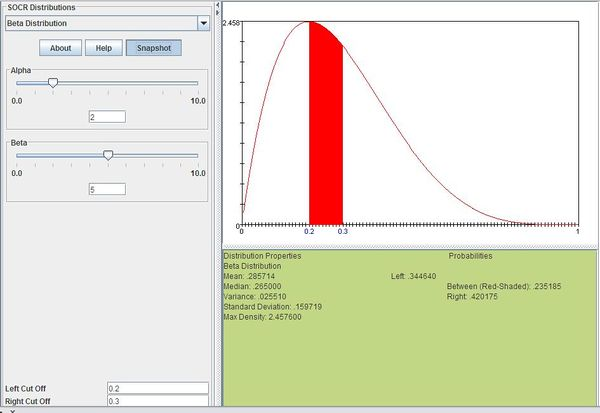# AP Statistics Curriculum 2007 Beta

## General Advance-Placement (AP) Statistics Curriculum - Beta Distribution

### Beta Distribution

Definition: Beta distribution is a distribution that models events which are constrained to take place within an interval defined by a minimum and maximum value.

Probability density function: For $$X\sim \operatorname{Beta}(\alpha,\beta)\!$$, the Beta probability density function is given by

$\frac{x^{\alpha-1}(1-x)^{\beta-1}}{\Beta(\alpha,\beta)}$

where

• $$\alpha$$ is a positive shape parameter
• $$\beta$$ is a positive shape parameter
• $$\textstyle\Beta(\alpha,\beta)=\int_0^1 t^{\alpha-1}(1-t)^{\beta-1}dt$$ or
$$\textstyle\Beta(\alpha,\beta)=\frac{\Gamma(x)\Gamma(y)}{\Gamma(x+y)}$$, where $$\Gamma(k)!=(k-1)!=1 \times 2 \times\ 3 \times\cdots \times (k-1)$$
• x is a random variable

Cumulative density function: Beta cumulative distribution function is given by

$\frac{\Beta_x(\alpha,\beta)}{\Beta(\alpha,\beta)}$

where

• $$\textstyle\Beta_x(\alpha,\beta)=\int_0^x t^{\alpha-1}(1-t)^{\beta-1}dt$$
• $$\textstyle\Beta(\alpha,\beta)=\int_0^1 t^{\alpha-1}(1-t)^{\beta-1}dt$$

Moment generating function: The Beta moment-generating function is

$M(t)=1+\sum_{k=1}^\infty (\prod_{r=0}^{k-1}\frac{\alpha+r}{\alpha+\beta+r})\frac{t^k}{k!}$

Expectation: The expected value of a Beta distributed random variable x is

$E(X)=\frac{\alpha}{\alpha+\beta}$

Variance: The Beta variance is

$Var(X)=\frac{\alpha\beta}{(\alpha+\beta)^2(\alpha+\beta+1)}$

### Applications

The Beta distribution is used in a range of disciplines including rule of succession, Bayesian statistics, and task duration modeling. Examples of events that may be modeled by Beta distribution include:

• The time it takes to complete a task
• The proportion of defective items in a shipment

### Example

Suppose that DVDs in a certain shipment are defective with a Beta distribution with $$\alpha=2$$ and $$\beta=5$$. Compute the probability that the shipment has 20% to 30% defective DVDs.

We can compute this as follows:

$P(0.2\le X\le 0.3)=\sum_{x=0.2}^{0.3}\frac{x^{2-1}(1-x)^{5-1}}{\Beta(2,5)}=0.235185$

The figure below shows this result using SOCR distributions"-----